# Natural numbers - 8th grade (13y) - math problems

#### Number of problems found: 157

• Unknown numbersThe sum of two consecutive natural numbers and their triple is 92. Find these numbers.
• Big numbersHow many natural numbers less than 10 to the sixth can be written in numbers: a) 9.8.7 b) 9.8.0
• AM of three numbersThe number 2010 can be written as the sum of 3 consecutive natural numbers. Determine the arithmetic mean of these numbers.
• Three-digitHow many three-digit natural numbers do not have the number 7?
• Count of roots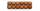How many solutions has equation x. y = 7757 with two unknowns on the set of natural numbers?
• Sum of sevenThe sum of seven consecutive odd natural numbers is 119. Determine the smallest of them.
• Three-digit integersHow many three-digit natural numbers exist that do not contain zero and are divisible by five?
• Twenty-fiveHow many three-digit natural numbers are divisible by 25?
• RectanglesHow many rectangles with area 8713 cm2 whose sides are natural numbers are?
• Two numbers 7The sum of two consecutive even numbers is 30. Find the numbers.
• How many 4How many 4 digit numbers that are divisible by 10 can be formed from the numbers 3, 5, 7, 8, 9, 0 such that no number repeats?
• Telephone numbers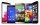How many 7-digit telephone numbers can we put together so that each number consists of different digits?
• Odd numbersThe sum of four consecutive odd numbers is 1048. Find those numbers ...
• Three-digit numbersWe have digits 0,1,4,7 that cannot be repeated. How many three-digit numbers can we write from them? You can help by listing all the numbers.
• Three numbersWe have three different non-zero digits. We will create all 3 digits numbers from them to use all 3 figures in each number. We add all the created numbers, and we get the sum of 1554. What were the numbers?
• How many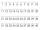How many double-digit numbers greater than 30 we can create from digits 0, 1, 2, 3, 4, 5? Numbers cannot be repeated in a two-digit number.
• Unknown number 716% of the unknown number is by 21 less than unknown number itself. Determine the natural unknown number.
• Even / odd numbers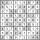a / Using variable n write two consecutive odd numbers. b / the sum of three consecutive odd numbers is 333. What are this numbers?
• A three-digit numbers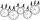Determine the total number of positive three-digit numbers that contain a digit 6.
• Drawing from a hatWhen drawing numbers from a hat from 1 to 35, we select random given numbers. What is the probability that the drawn numbers will be divisible by 8 and 2?

Do you have an interesting mathematical word problem that you can't solve it? Submit a math problem, and we can try to solve it.

We will send a solution to your e-mail address. Solved examples are also published here. Please enter the e-mail correctly and check whether you don't have a full mailbox.

Please do not submit problems from current active competitions such as Mathematical Olympiad, correspondence seminars etc...